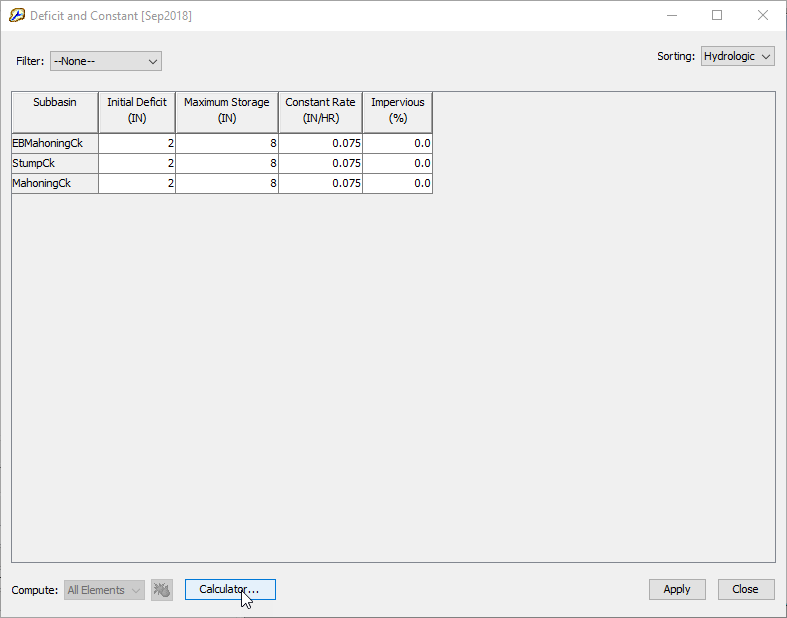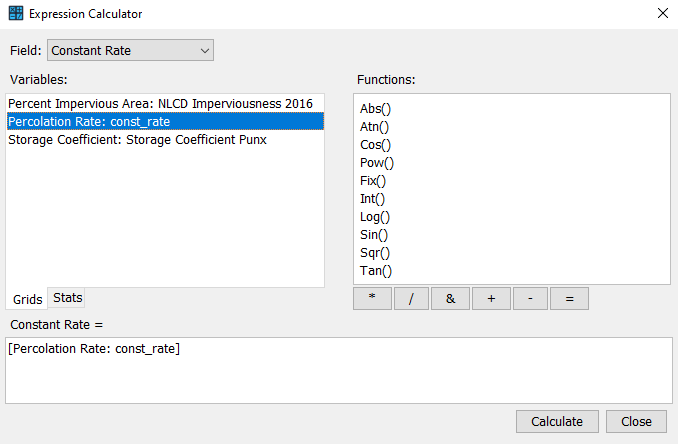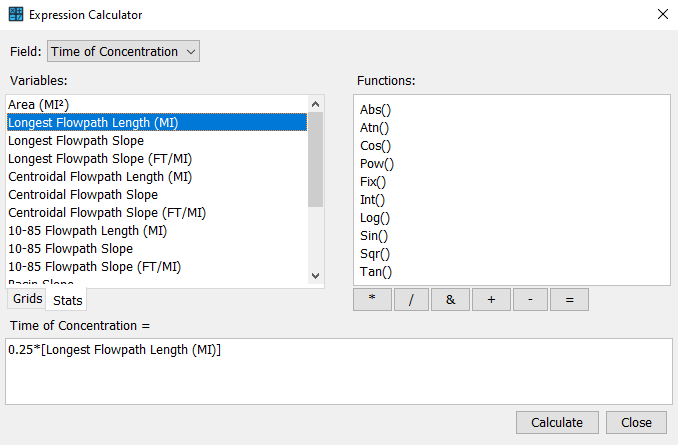Geospatial data is increasingly abundant in the earth science community and many hydrologic modeling parameters can be estimated from such data. An Expression Calculator is included in HEC-HMS to facilitate parameter estimation from GIS data. The Expression Calculator can be launched from basin global editors. Because many of the calculation options incorporate GIS processing, the Basin Model must include georeferenced subbasin elements for the Calculate button to be enabled.

The Expression Calculator is launched from the Calculator button of a Global Editor.Select the Parameter that you wish to calculate from the Field dropdown box in the Expression Calculator dialog. The selected parameter will appear above the Expression window as the left side of an mathematical expression as <Selected Parameter> =. In the Expression Calculator, grids and characteristics can be used as variables. In the Grids tab, you will see a list of user-imported gridded data. Double-click the desired Grid variable to add it the Expression window. When a grid is included in the expression, a zonal average value of the feature on the grid within the element is used in the calculation.

In the image below, a Percolation Rate grid has been included in the expression to estimate the Constant Rate parameter.The Stats tab displays a list of variables based on the physical characteristics of your watershed's subbasin or reach elements. When a characteristic is used in the expression, the characteristic value for a given feature (subbasin or reach element) is used in the calculation.  Double click on a variable to add it to the Expression window.

In the image below, the Longest Flowpath Length has been included in the expression to estimate the Time of Concentration parameter.Internal model variables for subbasin and reach elements are shown in the table below. Units (U.S. Customary or Metric) for the Stats variables correspond to the selection of the basin model's Unit System in the Component Editor.

Internal model variables for subbasin and reach elements

Stats Variables for SubbasinsUnitsStats Variables for ReachesUnits
AreaMI2 or KM2Reach LengthMI or KM
Longest Flowpath LengthMI or KMReach SlopeFT/MI, M/KM or dimensionless

Longest Flowpath Slope

FT/MI, M/KM or dimensionlessReach ReliefFT or M
Centroidal Flowpath SlopeFT/MI, M/KM or dimensionless

10-85 Flowpath LengthMI or KM

10-85 Flowpath SlopeFT/MI, M/KM or dimensionless

Basin SlopeFT/MI, M/KM or dimensionless

Basin ReliefFT or M

Drainage DensityMI/MI2 or KM/KM2

Elongation Ratiodimensionless

Relief RatioFT/MI, M/KM or dimensionless

The Functions window displays a list of Visual Basic (VBA) math functions that you can use in your expression. Below the functions window is a row of arithmetic operator buttons including multiplication (*), division (/), addition (+), subtraction (-) and string concatenation (&). You can use Grid variables, Stat variables, VBA functions, and the mathematical operators in a single expression to calculate an element's parameter value. Once the expression is complete. Click Calculate. The value of the selected parameter will be updated in the Global Editor Dialog.

The number of significant figures reported by the Expression Calculator can be controlled in Tools | Program Settings on the Compute tab. The program setting is called Expression Calculator Precision. The valid range for the setting is 1 to 5 significant figures. The program uses a default value of 3 unless modified by the user.